Waves Exam2 and Problem Solutions

Waves Exam2 and  Problem Solutions

1. Picture given below shows wave motion of source having frequency 2s-1.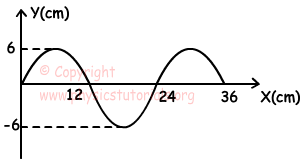a) Find wavelength

b) Velocity

c) Amplitude of wave.

a) Using picture given above, we find wavelength as;

24cm

b)

λ.f=V

24.2=V

V=48 cm/s

c) Using picture given above, we find amplitude as;
A=6 cm

2. Springs having different thicknesses are attached at point A. If the pulse having wavelength λ2 is transmitted pulse, find the relation between V1, V2, λ1 and λ2.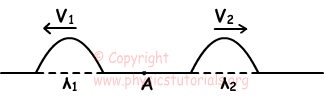Since both of the pulses have same shape, pulse comes from spring having thicker one to thinner one. Pulse having velocity V2 is transmitted wave, thus V1<V2.

V11.f

V22.f

λ12

3. If F is the focal point of curved obstacle, draw the reflected waves coming from wave source A.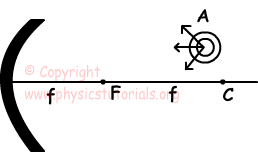Curved obstacle behaves like concave mirror. Image of incoming waves are located away from the center of the obstacle.4. Top view of water tank is given below. Draw the shapes of waves shown in the picture below in deep part of water tank.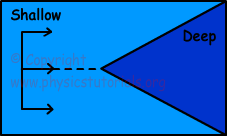Waves in deep part of water tank has larger velocity thus, shape of waves becomes;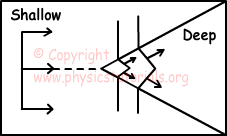5. Point A on wave becomes crest of wave after 3 second. Find period of wave.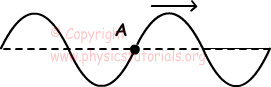Period of the wave;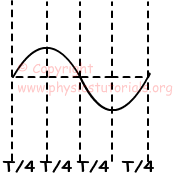3T/4=3s

T=4s

Author: